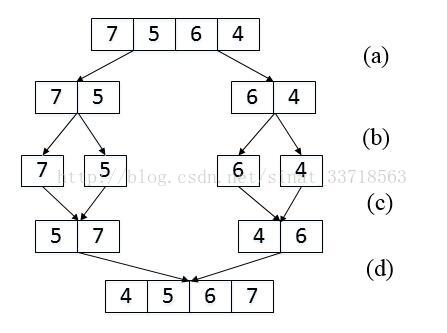## 题目6 第一次只出现一次的字符

### 代码

int FirstNotRepeatingChar(string str) { int asc={0}; int len=str.size(); if(len==0) return -1; for(int i=0;i<len;i++) asc[str[i]]++; for(int i=0;i<len;i++) { if(asc[str[i]]==1) return i; } return -1;}

## 题目7 丑数

### 代码1

bool isUgly(int number){ while(number%2==0) number/=2; while(number%3==0) number/=3; while(number%/5==0) number/=5; return (number==1)?true:false;}int GetUglyNumber(int index){ if(index<=0) return 0; int number=0; int uglyFound=0; while(uglyFound<index) { ++number; if(isUgly(number)) { uglyFound++; } } return number;}

## 题目8 数字在排序数组中出现的次数

### 代码

int GetNumberOfK(vector<int> data ,int k) { int len = data.size(); int count=0; for(int i=0;i<len;i++) { if(data[i]==k) ++count; } return count;}

## 题目9 两个链表的第一个公共节点

### 代码

 ListNode* FindFirstCommonNode( ListNode *pHead1, ListNode *pHead2) { int len1 = findListLenth(pHead1); int len2 = findListLenth(pHead2); //如果两个长度不相等，就把长的那个链表上的指针从开头往后移动|len1-len2|个位置，这样就相当于两个链表同一起跑线开始。 if(len1 > len2){ pHead1 = walkStep(pHead1,len1 - len2); }else{ pHead2 = walkStep(pHead2,len2 - len1); } //由于这个时候两个链表从指针所在位置往后长度肯定一致，所以这里指定pHead1和pHead2判断是否到尾部都可以 while(pHead1 != NULL){ //如果两个结点相等，自然就是第一个公共节点了，否则往后继续找 if(pHead1 == pHead2) return pHead1; pHead1 = pHead1->next; pHead2 = pHead2->next; } return NULL; } int findListLenth(ListNode *pHead1){ if(pHead1 == NULL) return 0; int sum = 1; while(pHead1 = pHead1->next) sum++; return sum; } ListNode* walkStep(ListNode *pHead1, int step){ while(step--){ pHead1 = pHead1->next; } return pHead1; }

## 题目10 数组中的逆序对

### 题目解析(a) 把长度为4的数组分解成两个长度为2的子数组；
(b) 把长度为2的数组分解成两个成都为1的子数组；
(c) 把长度为1的子数组 合并、排序并统计逆序对 ；
(d) 把长度为2的子数组合并、排序，并统计逆序对；

### 代码

int InversePairs(int* data, int length){ if(data==nullptr||length<0) return 0; int* copy=new int[length]; for(int i=0;i<length;++i) copy[i]=data[i]; int count=InversePairsCore(data,copy,0,length-1); delete[] copy; return count;}int InversePairsCore(int*data,int*copy,int start,int end){ if(start==end) { copy[start]=data[start]; return 0; } int length=(end-start)/2; int left=InversePairsCore(copy,data,start,start+length); int right=InversePairsCore(copy,data,start+length+1,end); //i初始化为前半段最后一个数字的下标 int i=start+length; //j初始化为后半段最后一个数字的下标 int j=end; int indexCopy=end; int count=0; while(i>=start&&j>=start+length+1) { if(data[i]>data[j]) { copy[indexCopy--]=data[i--]; count+=j-start-length; } else { copy[indexCopy--]=data[j--]; } } for(;i>=start;--i) copy[indexCopy--]=data[i]; for(;j>=start+length+1;--j) copy[indexCopy--]=data[j]; return left+right+count;}